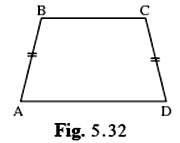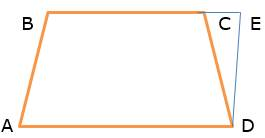SSC BOARD PAPERS IMPORTANT TOPICS COVERED FOR BOARD EXAM 2024

### Practice Set 5.4, Chapter 5 - Quadrilaterals Mathematics Part II Solutions for Class 9 Math

##### Class 9th Mathematics Part Ii MHB Solution

###### Practice Set 5.4
Question 1.

In 􀀍IJKL, side IJ || side KL ∠I = 108° ∠K = 53° then find the measures of ∠J and ∠L.

IJ ∥ KL and IL is transversal

∠I + ∠L = 180° (adjacent angles on the same side of the transversal)

⇒ ∠L = 180° – 108° = 72°

Now again IJ ∥ KL and JK is transversal

∠J + ∠K= 180 ° (adjacent angles on the same side of the transversal)

⇒ ∠K = 180° – 53° = 127°

Question 2.

In 􀀍ABCD, side BC || side AD, side AB ≅ sided DC If ∠A = 72° then find the measures of ∠B, and ∠D.

⇒ the quadrilateral is a parallelogram (pair of opposite sides are equal and parallel)

∠ A = 72°

⇒ ∠C = 72° (opposite angles of parallelogram are congruent)

∠B = 180° – 72° = 108° (adjacent angles of a parallelogram are supplementary)

∠D = 108° (opposite angles of parallelogram are congruent)

Question 3.

In 􀀍ABCD, side BC < side AD (Figure 5.32) side BC || side AD and if side BE ≅ side CD then prove that ∠ABC ≅ ∠DCB.The figure of the question is given below:Construction: we will draw a segment ∥ to BA meeting BC in E through point D.

And AB ∥ ED (construction)

⇒ AB = DE (distance between parallel lines is always same)

Hence ABDE is parallelogram

⇒ ∠ABE ≅ ∠DEC (corresponding angles on the same side of transversal)

And segBA ≅ seg DE (opposite sides of a ∥gram)

But given BA ≅ CD

So seg DE ≅ seg CD

⇒∠CED ≅ ∠DCE ( ∵ Δ CED is isosceles with CE = CD)

(Angle opposite to opposite sides are equal)

⇒ ∠ABC ≅ ∠DCB